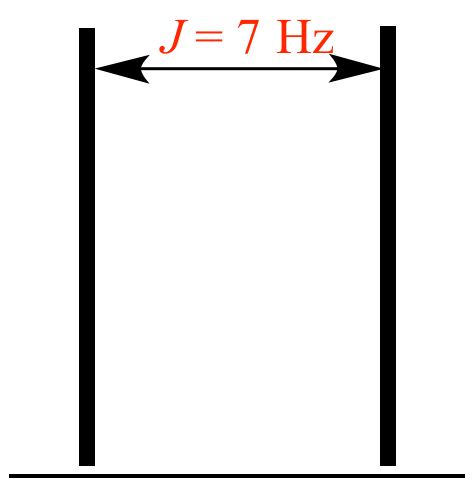Illustrated Glossary of Organic Chemistry

J (J): (1) The joule. An International System of Units energy unit equal to an applied force of one newton through a distance of one meter, or the energy equivalent to passing an electric current of one ampere through a resistance of one ohm for one second. When used as an energy unit, J is not italicized, for example, 16 kJ (16 kiloJoules).

(2) In NMR spectroscopy, coupling constant. When denoting a coupling constant J is italicized, for example J = 7 Hz.The distance between the lines in this doublet is 7 Hz, so J = 7 Hz.